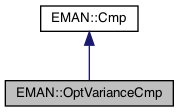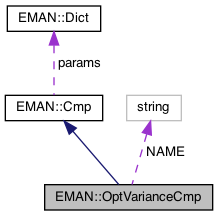EMAN2
EMAN::OptVarianceCmp Class Reference

Variance between two data sets after various modifications. More...

`#include <cmp.h>`

Inheritance diagram for EMAN::OptVarianceCmp:[legend]
Collaboration diagram for EMAN::OptVarianceCmp:[legend]

## Public Member Functions

OptVarianceCmp ()

float cmp (EMData *image, EMData *with) const
To compare 'image' with another image passed in through its parameters. More...

string get_name () const
Get the Cmp's name. More...

string get_desc () const

TypeDict get_param_types () const
Get Cmp parameter information in a dictionary. More...

float get_scale () const

float get_shift () constPublic Member Functions inherited from EMAN::Cmp
virtual ~Cmp ()

virtual Dict get_params () const
Get the Cmp parameters in a key/value dictionary. More...

virtual void set_params (const Dict &new_params)
Set the Cmp parameters using a key/value dictionary. More...

static CmpNEW ()

## Static Public Attributes

static const string NAME = "optvariance"

## Private Attributes

float scale

float shiftProtected Member Functions inherited from EMAN::Cmp
void validate_input_args (const EMData *image, const EMData *with) constProtected Attributes inherited from EMAN::Cmp
Dict params

## Detailed Description

Variance between two data sets after various modifications.

Generally, 'this' should be noisy and 'with' should be less noisy. linear scaling (mx + b) of the densities in 'this' is performed to produce the smallest possible variance between images.

If keepzero is set, then zero pixels are left at zero (b is not added). if matchfilt is set, then 'with' is filtered so its radial power spectrum matches 'this' If invert is set, then (y-b)/m is applied to the second image rather than mx+b to the first.

To modify 'this' to match the operation performed here, scale should be applied first, then b should be added

Definition at line 575 of file cmp.h.

## ◆ OptVarianceCmp()

 EMAN::OptVarianceCmp::OptVarianceCmp ( )
inline

Definition at line 578 of file cmp.h.

578: scale(0), shift(0) {}

Referenced by NEW().

## ◆ cmp()

 float OptVarianceCmp::cmp ( EMData * image, EMData * with ) const
virtual

To compare 'image' with another image passed in through its parameters.

An optional transformation may be used to transform the 2 images.

Parameters
 image The first image to be compared. with The second image to be comppared.
Returns
The comparison result. Smaller better by default

Implements EMAN::Cmp.

Definition at line 1036 of file cmp.cpp.

1037{
1038 ENTERFUNC;
1039 validate_input_args(image, with);
1040
1041 int keepzero = params.set_default("keepzero", 1);
1042 int invert = params.set_default("invert",0);
1043 int matchfilt = params.set_default("matchfilt",1);
1044 int matchamp = params.set_default("matchamp",0);
1046 int dbug = params.set_default("debug",0);
1047
1048 size_t size = (size_t)image->get_xsize() * image->get_ysize() * image->get_zsize();
1049
1050
1051 EMData *with2=NULL;
1052 if (matchfilt) {
1053 EMData *a = image->do_fft();
1054 EMData *b = with->do_fft();
1055
1058
1059 float avg=0;
1060 for (size_t i=0; i<a->get_ysize()/2.0f; i++) {
1061 rfa[i]=(rfb[i]==0?0.0f:(rfa[i]/rfb[i]));
1062 avg+=rfa[i];
1063 }
1064
1065 avg/=a->get_ysize()/2.0f;
1066 for (size_t i=0; i<a->get_ysize()/2.0f; i++) {
1067 if (rfa[i]>avg*10.0) rfa[i]=10.0; // If some particular location has a small but non-zero value, we don't want to overcorrect it
1068 }
1069 rfa=0.0;
1070
1071 if (dbug) b->write_image("a.hdf",-1);
1072
1074 with2=b->do_ift();
1075
1076 if (dbug) b->write_image("a.hdf",-1);
1077 if (dbug) a->write_image("a.hdf",-1);
1078
1079/* if (dbug) {
1080 FILE *out=fopen("a.txt","w");
1081 for (int i=0; i<a->get_ysize()/2.0; i++) fprintf(out,"%d\t%f\n",i,rfa[i]);
1082 fclose(out);
1083
1084 out=fopen("b.txt","w");
1085 for (int i=0; i<a->get_ysize()/2.0; i++) fprintf(out,"%d\t%f\n",i,rfb[i]);
1086 fclose(out);
1087 }*/
1088
1089
1090 delete a;
1091 delete b;
1092
1093 if (dbug) {
1094 with2->write_image("a.hdf",-1);
1095 image->write_image("a.hdf",-1);
1096 }
1097
1098// with2->process_inplace("matchfilt",Dict("to",this));
1099// x_data = with2->get_data();
1100 }
1101
1102 // This applies the individual Fourier amplitudes from 'image' and
1103 // applies them to 'with'
1104 if (matchamp) {
1105 EMData *a = image->do_fft();
1106 EMData *b = with->do_fft();
1107 size_t size2 = (size_t)a->get_xsize() * a->get_ysize() * a->get_zsize();
1108
1109 a->ri2ap();
1110 b->ri2ap();
1111
1113 float * bd=b->get_data();
1114
1115 for (size_t i=0; i<size2; i+=2) bd[i]=ad[i];
1116 b->update();
1117
1118 b->ap2ri();
1119 with2=b->do_ift();
1120//with2->write_image("a.hdf",-1);
1121 delete a;
1122 delete b;
1123 }
1124
1125 const float * x_data;
1126 if (with2) x_data=with2->get_const_data();
1127 else x_data = with->get_const_data();
1128 const float *const y_data = image->get_const_data();
1129
1130 size_t nx = image->get_xsize();
1131 float m = 0;
1132 float b = 0;
1133
1134 // This will write the x vs y file used to calculate the density
1135 // optimization. This behavior may change in the future
1136 if (dbug) {
1137 FILE *out=fopen("dbug.optvar.txt","w");
1138 if (out) {
1139 for (size_t i=0; i<size; i++) {
1140 if ( !keepzero || (x_data[i] && y_data[i])) fprintf(out,"%g\t%g\n",x_data[i],y_data[i]);
1141 }
1142 fclose(out);
1143 }
1144 }
1145
1146
1147 Util::calc_least_square_fit(size, x_data, y_data, &m, &b, keepzero);
1148 if (m == 0) {
1149 m = FLT_MIN;
1150 }
1151 b = -b / m;
1152 m = 1.0f / m;
1153
1154 // While negative slopes are really not a valid comparison in most cases, we
1155 // still want to detect these instances, so this if is removed
1156/* if (m < 0) {
1157 b = 0;
1158 m = 1000.0;
1159 }*/
1160
1161 double result = 0;
1162 int count = 0;
1163
1165 if (image->get_zsize()!=1) throw ImageDimensionException("radweight option is 2D only");
1166 if (keepzero) {
1167 for (size_t i = 0,y=0; i < size; y++) {
1168 for (size_t x=0; x<nx; i++,x++) {
1169 if (y_data[i] && x_data[i]) {
1170 if (invert) result += Util::square(x_data[i] - (y_data[i]-b)/m)*(hypot((float)x,(float)y)+nx/4.0);
1171 else result += Util::square((x_data[i] * m) + b - y_data[i])*(hypot((float)x,(float)y)+nx/4.0);
1172 count++;
1173 }
1174 }
1175 }
1176 result/=count;
1177 }
1178 else {
1179 for (size_t i = 0,y=0; i < size; y++) {
1180 for (size_t x=0; x<nx; i++,x++) {
1181 if (invert) result += Util::square(x_data[i] - (y_data[i]-b)/m)*(hypot((float)x,(float)y)+nx/4.0);
1182 else result += Util::square((x_data[i] * m) + b - y_data[i])*(hypot((float)x,(float)y)+nx/4.0);
1183 }
1184 }
1185 result = result / size;
1186 }
1187 }
1188 else {
1189 if (keepzero) {
1190 for (size_t i = 0; i < size; i++) {
1191 if (y_data[i] && x_data[i]) {
1192 if (invert) result += Util::square(x_data[i] - (y_data[i]-b)/m);
1193 else result += Util::square((x_data[i] * m) + b - y_data[i]);
1194 count++;
1195 }
1196 }
1197 result/=count;
1198 }
1199 else {
1200 for (size_t i = 0; i < size; i++) {
1201 if (invert) result += Util::square(x_data[i] - (y_data[i]-b)/m);
1202 else result += Util::square((x_data[i] * m) + b - y_data[i]);
1203 }
1204 result = result / size;
1205 }
1206 }
1207 scale = m;
1208 shift = b;
1209
1210 image->set_attr("ovcmp_m",m);
1211 image->set_attr("ovcmp_b",b);
1212 if (with2) delete with2;
1213 EXITFUNC;
1214
1215#if 0
1216 return (1 - result);
1217#endif
1218
1219 return static_cast<float>(result);
1220}
void validate_input_args(const EMData *image, const EMData *with) const
Definition: cmp.cpp:81
Dict params
Definition: cmp.h:132
type set_default(const string &key, type val)
Default setting behavior This can be achieved using a template - d.woolford Jan 2008 (before there wa...
Definition: emobject.h:569
EMData stores an image's data and defines core image processing routines.
Definition: emdata.h:82
void apply_radial_func(float x0, float dx, vector< float >array, bool interp=true)
multiplies by a radial function in fourier space.
Definition: emdata.cpp:2677
vector< float > calc_radial_dist(int n, float x0, float dx, int inten)
Definition: emdata.cpp:2781
static void calc_least_square_fit(size_t nitems, const float *data_x, const float *data_y, float *p_slope, float *p_intercept, bool ignore_zero, float absmax=0)
calculate the least square fit value.
Definition: util.cpp:538
static int square(int n)
Calculate a number's square.
Definition: util.h:736
#define ImageDimensionException(desc)
Definition: exception.h:166
#define ENTERFUNC
Definition: log.h:48
#define EXITFUNC
Definition: log.h:49
#define y(i, j)
Definition: projector.cpp:1516
#define x(i)
Definition: projector.cpp:1517

## ◆ get_desc()

 string EMAN::OptVarianceCmp::get_desc ( ) const
inlinevirtual

Implements EMAN::Cmp.

Definition at line 587 of file cmp.h.

588 {
589 return "Real-space variance after density optimization, self should be noisy and target less noisy. Linear transform applied to density to minimize variance.";
590 }

## ◆ get_name()

 string EMAN::OptVarianceCmp::get_name ( ) const
inlinevirtual

Get the Cmp's name.

Each Cmp is identified by a unique name.

Returns
The Cmp's name.

Implements EMAN::Cmp.

Definition at line 582 of file cmp.h.

583 {
584 return NAME;
585 }
static const string NAME
Definition: cmp.h:619

References NAME.

## ◆ get_param_types()

 TypeDict EMAN::OptVarianceCmp::get_param_types ( ) const
inlinevirtual

Get Cmp parameter information in a dictionary.

Each parameter has one record in the dictionary. Each record contains its name, data-type, and description.

Returns
A dictionary containing the parameter info.

Implements EMAN::Cmp.

Definition at line 597 of file cmp.h.

598 {
599 TypeDict d;
600 d.put("invert", EMObject::INT, "If set, 'with' is rescaled rather than 'this'. 'this' should still be the noisier image. (default=0)");
601 d.put("keepzero", EMObject::INT, "If set, zero pixels will not be adjusted in the linear density optimization. (default=1)");
602 d.put("matchfilt", EMObject::INT, "If set, with will be filtered so its radial power spectrum matches 'this' before density optimization of this. (default=1)");
603 d.put("matchamp", EMObject::INT, "Takes per-pixel Fourier amplitudes from self and imposes them on the target, but leaves the phases alone. (default=0)");
604 d.put("radweight", EMObject::INT, "Upweight variances closer to the edge of the image. (default=0)");
605 d.put("debug", EMObject::INT, "Performs various debugging actions if set.");
606 return d;
607 }

References EMAN::EMObject::INT, and EMAN::TypeDict::put().

## ◆ get_scale()

 float EMAN::OptVarianceCmp::get_scale ( ) const
inline

Definition at line 609 of file cmp.h.

610 {
611 return scale;
612 }

References scale.

## ◆ get_shift()

 float EMAN::OptVarianceCmp::get_shift ( ) const
inline

Definition at line 614 of file cmp.h.

615 {
616 return shift;
617 }

References shift.

## ◆ NEW()

 static Cmp * EMAN::OptVarianceCmp::NEW ( )
inlinestatic

Definition at line 592 of file cmp.h.

593 {
594 return new OptVarianceCmp();
595 }

References OptVarianceCmp().

## ◆ NAME

 const string OptVarianceCmp::NAME = "optvariance"
static

Definition at line 619 of file cmp.h.

Referenced by get_name().

## ◆ scale

 float EMAN::OptVarianceCmp::scale
mutableprivate

Definition at line 622 of file cmp.h.

Referenced by cmp(), and get_scale().

## ◆ shift

 float EMAN::OptVarianceCmp::shift
mutableprivate

Definition at line 623 of file cmp.h.

Referenced by cmp(), and get_shift().

The documentation for this class was generated from the following files: